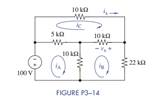Chat Now

# Formulate mesh-current equations for the circuit in Figure P3−14. Arrange the results in...

### Formulate mesh-current equations for the circuit in Figure P3−14. Arrange the results in...

(a) Formulate mesh-current equations for the circuit in Figure P3−14. Arrange the results in matrix form Ax = b.

(b) Solve for iA, iB, and iC.

(c) Use these results to find vand ix.manish jayant 28-Jul-2020Get solution